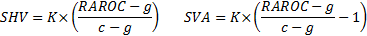/

### Enterprise Risk Management Formula Book 13. Miscellaneous

13.1        Combining solvency capital requirements using correlations

A correlation based combination of individual solvency capital requirements involves a formula along the lines of: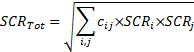13.2        Credit risk modelling

A single factor credit portfolio model generally assumes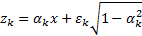where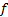is the (standardised) factor return/movement,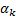is the exposure of the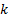’th obligor to that factor and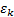is the idiosyncratic noise term for that obligor.

If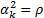is the same for all instruments (e.g. all are assumed to have same correlation with market plus only an idiosyncratic term) then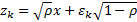. In such circumstances and if all obligors have the same probability of default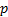say then probability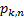thatout ofdefault is: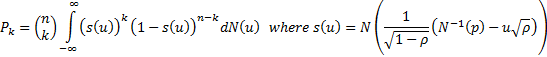If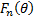is the cumulative probability that the percentage loss on the portfolio does not exceed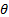then in the well diversified limit (Vasicek’s loss distribution):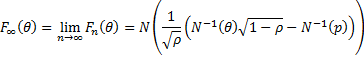13.3        GARCH models

Risk models may cater for heteroscedasticity by including GARCH features, e.g. the model might involve formulae along the lines of (in practice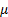will slowly evolve as additional data is received):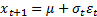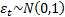where for, say, a GARCH(1,1) model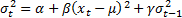RiskMetrics typically uses the following approach for estimating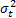(often using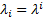for some suitably chosen decay factor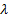) which can be viewed as an example of a GARCH approach and/or using weighted moments.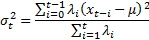13.4        Linear algebra and principal components

Suppose we have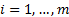data series (e.g. returns) each with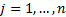observations,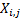, that are coincident in time across the different data series. Suppose the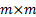covariance matrix of the (empirical) covariances between the different series is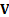. The eigenvalues and eigenvectors of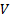are the values of(scalar) and associated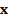(vector) for which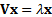. Anmatrix has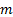(not necessarily distinct) eigenvalues and associated eigenvectors. Eigenvectors associated with distinct eigenvalues are orthogonal, i.e.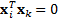for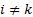. Orthonormal eigenvectors have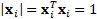andfor.  For any distinct eigenvalue the associated orthonormal eigenvector is unique up to a change of sign. If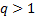eigenvalues all take the same value then it is possible to find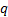orthogonal eigenvectors corresponding to all of these eigenvalues. For empirical covariance matrices,is symmetric non-negative definite (and positive definite if no two data series are perfectly correlated) and all of itseigenvalues,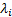, are greater than or equal to zero. One way of telling if a matrix is positive definite is to test whether it is possible to apply a Cholesky decomposition to it.

The eigenvalues and associated eigenvectors of an empirical covariance matrix may be sorted so that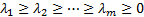. The first principal component is the mixture of the underlying (de-meaned) series, i.e. the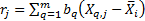, that corresponds to the orthonormal eigenvector,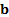, corresponding to the largest eigenvalue of. This choice ofmaximises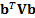subject to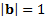,  Other (lesser) principal components correspond to orthonormal eigenvectors corresponding to smaller eigenvalues.

13.5        Central limit theorem

Suppose we have a series of independent random variables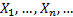each with finite (bounded) expected value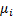and finite (bounded) standard deviation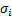. Suppose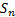and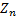are defined as: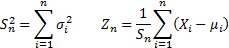Then subject to certain regularity conditions the distribution oftends asymptotically to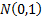(it is exactlyif each of the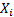is normally distributed).

13.6        Cornish-Fisher asymptotic expansion

The (4th moment) Cornish-Fisher asymptotic expansion approximates a standardised QQ-plot via the following function: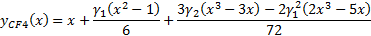where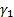and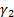are the skew and excess kurtosis of the data.

13.7        Euler capital allocation principle

A function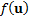where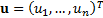is said to be homogenous of orderif: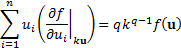Suppose we havebusiness lines, the outcome (loss) to each business line given its current size is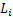(a random variable) so the total loss is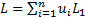where for the current business portfolio the business line allocation is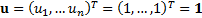. Suppose the risk measure used to determine economic capital is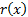and that it is homogeneous of order 1, i.e.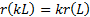. Then the Euler capital allocation principle (and, in effect, the Marginal VaR or Internal beta approach to setting RAROC rates) allocates total economic capital,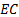(technically a function of the business portfolio allocation,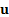) into capital for each business line,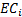, as follows:13.8        Equiprobable outcomes for a multivariate normal distribution

If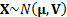where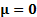then equiprobable scenarios (i.e. contours where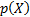is constant) are ellipsoids defined by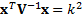for some constant value of. The probability that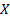lies within this ellipsoid is given by a chi-squared withdegrees of freedom: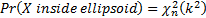13.9        RAROC, EIC, SHV and SVA

Risk adjusted return on capital (RAROC) is usually defined as follows, where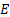= Adjusted earnings = Earnings – Interest costexpected lossfunding costother costs and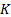= capital: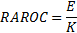Economic income created (EIC) is usually defined as where= per unit cost of equity (i.e. hurdle rate):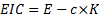Shareholder value (SHV) and shareholder value added (SVA) (also known as economic value added, EVA) translate current period return contribution to overall economic value. Given suitable assumptions about future growth prospects for a business,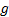, these are: